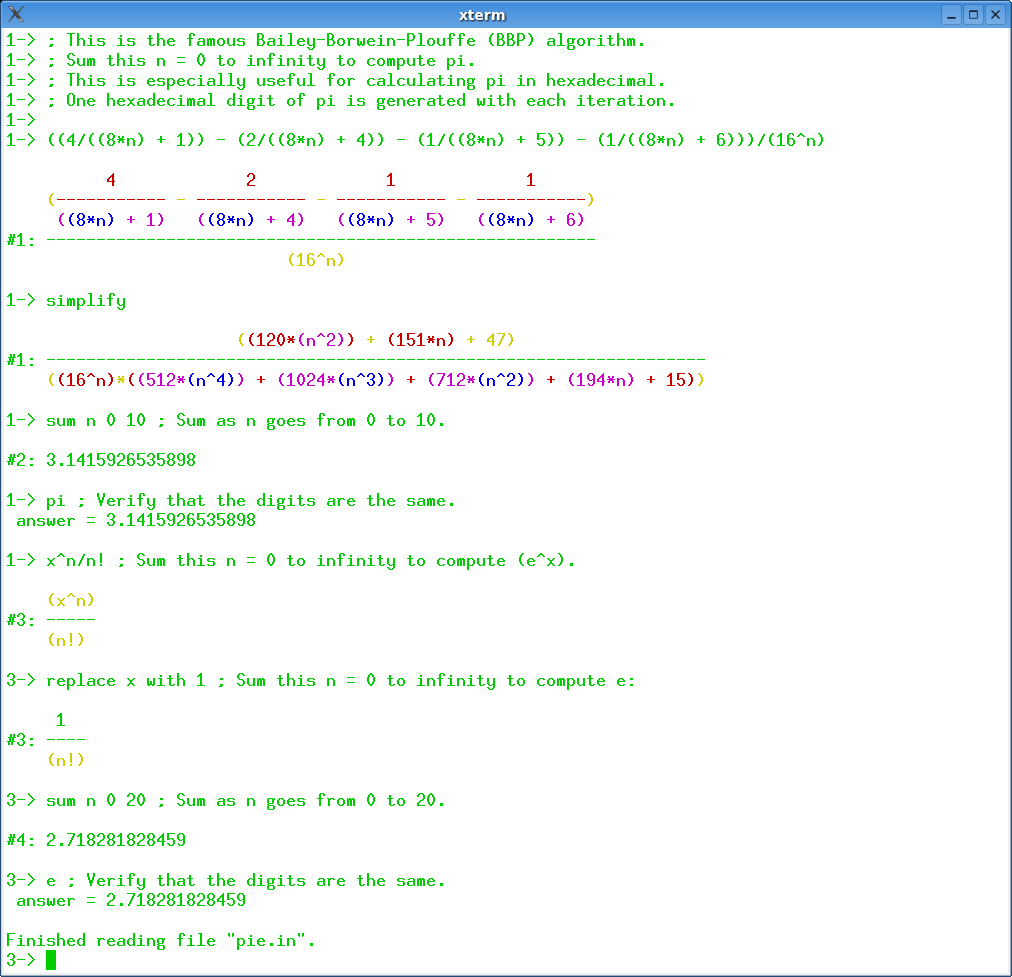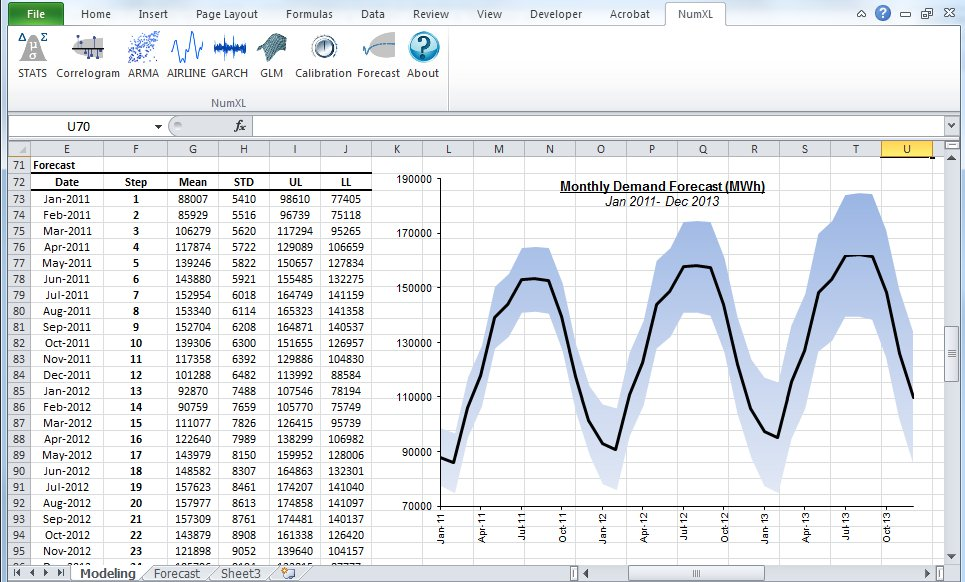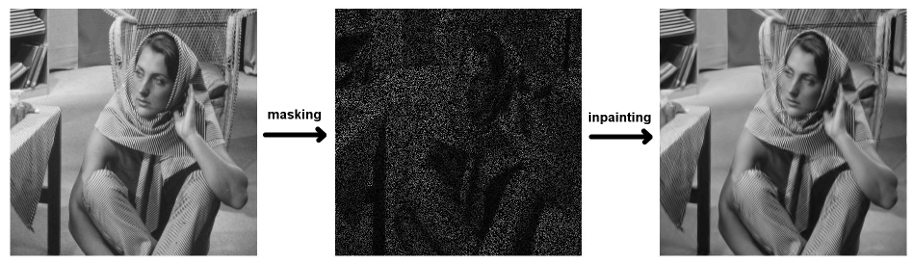## 24 Search Results

### Axiom

Axiom is a general purpose Computer Algebra system. It is useful for research and development of mathematical algorithms. It defines a strongly typed, mathematically correct type hierarchy. It has a programming language and a built-in compiler.

### CASA

CASA is a special-purpose system for computational algebra and constructive algebraic geometry. The system has been developed since 1990. CASA is the ongoing product of the Computer Algebra Group at the Research Institute for Symbolic Computation (RISC-Linz), the University of Linz, Austria, under the direction of Prof. Winkler. The system is built on the kernel of the widely used computer algebra system Maple.

### Cinderella

Cinderella is a software system for doing geometry on a computer. The new version Cinderella.2 also includes physics simulations and algorithmic elements.

### GAP

GAP is a system for computational discrete algebra, with particular emphasis on Computational Group Theory. GAP provides a programming language, a library of thousands of functions implementing algebraic algorithms written in the GAP language as well as large data libraries of algebraic objects. GAP is used in research and teaching for studying groups and their representations, rings, vector spaces, algebras, combinatorial structures, and more. GAP is developed by international cooperation. The system, including source, is distributed freely under the terms of the GNU General Public License. You can study and easily modify or extend GAP for your special use. The current version is GAP 4, the older version GAP 3 is still available.

### GELDA

GELDA is a Fortran77 software package for the numerical integration of general linear differential-algebraic equations (DAE) with variable coefficients of arbitrary index. The implementation of GELDA is based on the construction of the discretization scheme, which first determines all the local invariants and then transforms the linear DAE into an equivalent strangeness-free DAE with the same solution set. The resulting strangeness-free system is allowed to have nonuniqueness in the solution set or inconsistency in the initial values or inhomogeneities. In the case that the DAE is found to be uniquely solvable, GELDA is able to compute a consistent initial value and apply the well-known integration schemes for DAEs. In GELDA the BDF methods and Runge-Kutta schemes are implemented.

### IBM SPSS Statistics

With IBM SPSS Statistics, you can get a quick look at your data, formulate hypotheses for additional testing, and then carry out a number of procedures to help clarify relationships between variables, create clusters, identify trends and make predictions.

### KnotPlot

KnotPlot is a program to visualize and manipulate knots in three and four dimensions. Knots can be loaded from a database of almost 1000 knots and links or sketched by hand in three dimensions. Also, knots may be constructed via the Conway notation or using a tangle calculator. A number of special knot types (torus knots, knot chains, Lissajous knots) may be created on the fly. Finally, new knots can be created from old knots using a number of transformations.

### LiE

LiE is the name of a software package that enables mathematicians and physicists to perform computations of a Lie group theoretic nature. It focuses on the representation theory of complex semisimple (reductive) Lie groups and algebras, and on the structure of their Weyl groups and root systems. LiE does not compute directly with elements of the Lie groups and algebras themselves; it rather computes with weights, roots, characters and similar objects.

### Maple

Maple is an environment for scientific and engineering problem-solving, mathematical exploration, data visualization and technical authoring.

More information### Mathematica

Mathematica seamlessly integrates a numeric and symbolic computational engine, graphics system, programming language, documentation system, and advanced connectivity to other applications.

### Mathomatic

Mathomatic is a free, portable, general-purpose CAS (Computer Algebra System) and calculator software that can symbolically solve, simplify, combine, and compare equations, perform complex number and polynomial arithmetic, etc. It does some calculus and is very easy to use.

More information### Maxima

Maxima is a system for the manipulation of symbolic and numerical expressions, including differentiation, integration, Taylor series, Laplace transforms, ordinary differential equations, systems of linear equations, and vectors, matrices, and tensors. Maxima produces high precision results by using exact fractions and arbitrarily long floating point representations, and can plot functions and data in two and three dimensions.

MuPAD is a mathematical expert system for doing symbolic and exact algebraic computations as well as numerical calculations with almost arbitrary accuracy. For example, the number of significant digits can be chosen freely. Apart from a vast variety of mathematical libraries the system provides tools for high quality visualization of 2- and 3-dimensional objects. On Microsoft Windows, Apple Macintosh and Linux systems, MuPAD offers a flexible notebook concept for creating mathematical documents combining texts, graphics, formulas, computations and mathematical visualizations and animations. On Microsoft Windows MuPAD further supports the technologies OLE, ActiveX Automation, DCOM, RTF and HTML. Thus it offers a natural integration in Office applications like Word or PowerPoint as well as others.

### NumXL

NumXL is a suite of time series Excel add-ins. It transforms your Microsoft Excel application into a first-class time series software and econometrics tool, offering the kind of statistical accuracy offered by the leading statistical packages. NumXL integrates natively with Excel, adding scores of econometric functions, a rich set of shortcuts, and intuitive user interfaces to guide you through the entire process.

More information### Octave

GNU Octave is a high-level language, primarily intended for numerical computations. It provides a convenient command line interface for solving linear and nonlinear problems numerically, and for performing other numerical experiments using a language that is mostly compatible with Matlab. It may also be used as a batch-oriented language. Octave has extensive tools for solving common numerical linear algebra problems, finding the roots of nonlinear equations, integrating ordinary functions, manipulating polynomials, and integrating ordinary differential and differential-algebraic equations. It is easily extensible and customizable via user-defined functions written in Octave's own language, or using dynamically loaded modules written in C++, C, Fortran, or other languages.

### PLTMG

PLTMG is a package for solving elliptic partial differential equations in general regions of the plane. It is based on continuous piecewise linear triangular finite elements, and features adaptive local mesh refinement, multigraph iteration, and pseudo-arclength continuation options for parameter dependencies. It also provides options for solving several classes of optimal control and obstacle problems. The package includes an initial mesh generator and several graphics packages. Support for the Bank-Holst parallel adaptive meshing strategy is also provided. PLTMG is provided as Fortran (and a little C) source code, in both single and double precision versions. The code has interfaces to X-Windows, MPI, and Michael Holst's OpenGL image viewer SG. The X-Windows, MPI, and SG interfaces require libraries that are NOT provided as part of the PLTMG package.

### polymake

polymake is an object-oriented system for experimental discrete mathematics. The typical working cycle of a polymake user starts with the construction of an object of interest, auch as a convex polytope, a finite simplicial complex, a graph, etc. It is then possible to ask the system for some of the object's properties or for some form of visualization. Further steps might include more elaborate constructions based on previously defined objects. Each class of polymake objects comes with a set of rules which describe how a new property of an object can be derived from previously known ones. It is a key feature that the user can extend or modify the set of rules, add further properties or even new classes of objects (with entirely new rule bases). The functions provided include: several convex hull algorithms, face lattices of convex polytopes, Voronoi diagrams and Delaunay decompositions (in arbitrary dimensions), simplicial homology (with integer coefficients), simplicial cup and cap products, intersection forms of triangulated 4-manifolds. Several forms of (interactive) visualization via interfaces to Geomview, JavaView and other programs.

### rbMIT

The rbMIT © MIT software package implements in Matlab® all the general reduced basis algorithms. The rbMIT © MIT software package is intended to serve both (as Matlab® source) "Developers" — numerical analysts and computational tool-builders — who wish to further develop the methodology, and (as Matlab® "executables") "Users" — computational engineers and educators — who wish to rapidly apply the methodology to new applications. The rbMIT software package was awarded with the Springer Computational Science and Engineering Prize in 2009.

### Sage

SAGE is a framework for number theory, algebra, and geometry computation. It is open source and freely available under the terms of the GNU General Public License (GPL). SAGE is a Python library with a customized interpreter. It is written in Python, C++, and C (via Pyrex). Python (http://www.python.org) is an open source object-oriented interpreted language, with a large number of libraries, e.g., for numerical analysis, which are available to users of SAGE. Python can also be accessed in library mode from C/C++ programs. SAGE provides an interface to several important open source libraries, including Cremona’s MWRANK library for computing with elliptic curves, the PARI library (pari.math.u-bordeaux.fr) for number theory, Shoup’s number theory library NTL (http://www.shoup.net/ntl/), SINGULAR (http://www.singular.uni-kl.de) for commutative algebra, GAP (http://www.gap-system.org) for group theory and combinatorics, and maxima (http://maxima.sourceforge.net) for symbolic computation and calculus.

More information### Scilab

Scilab is a numerical computation system similiar to Matlab or Simulink. Scilab includes hundreds of mathematical functions, and programs from various languages (such as C or Fortran) can be added interactively. It has sophisticated data structures (including lists, polynomials, rational functions, and linear systems), an interpreter, and a high-level programming language. Scilab has been designed to be an open system where the user can define new data types and operations on these data types by using overloading. A number of toolboxes are available with the system.

### Scilab Image Processing Toolbox

SIP provides image processing, pattern recognition, and computer vision routines for SciLab, a Matlab-like matrix-oriented programming environment. SIP is able to read/write images in almost 90 major formats, including JPEG, PNG, BMP, GIF, FITS, and TIFF. It includes routines for filtering, segmentation, edge detection, morphology, curvature, fractal dimension, distance transforms, multiscale skeletons, and more.

### ShearLab 3D

ShearLab 3D is a MATLAB Library, developed for processing two- and three-dimensional signals using a certain class of basis functions named shearlets. These functions were developed with the goal of constructing systems of basis-functions nicely suited for representing anisotropic features (e.g. curvilinear singularities) that often are present in multivariate data. ShearLab 3D is optimized for GPU computing with CUDA and contains examples for applications like image and video denoising or image and video inpainting.

More information### SINGULAR

SINGULAR is a Computer Algebra system for polynomial computations in commutative algebra, algebraic geometry, and singularity theory. SINGULAR's main computational objects are ideals and modules over a large variety of baserings. The baserings are polynomial rings over a field (e.g., finite fields, the rationals, floats, algebraic extensions, transcendental extensions), or localizations thereof, or quotient rings with respect to an ideal. SINGULAR features fast and general implementations for computing Groebner and standard bases, including e.g. Buchberger's algorithm and Mora's Tangent Cone algorithm. Furthermore, it provides polynomial factorizations, resultant, characteristic set and gcd computations, syzygy and free-resolution computations, and many more related functionalities. Based on an easy-to-use interactive shell and a C-like programming language, SINGULAR's internal functionality is augmented and user-extendible by libraries written in the SINGULAR programming language. A general and efficient implementation of communication links allows SINGULAR to make its functionality available to other programs.